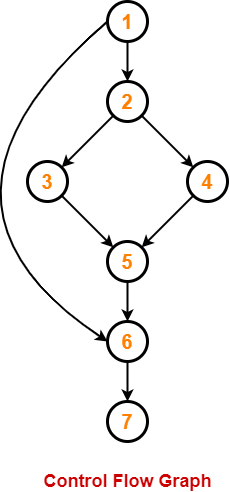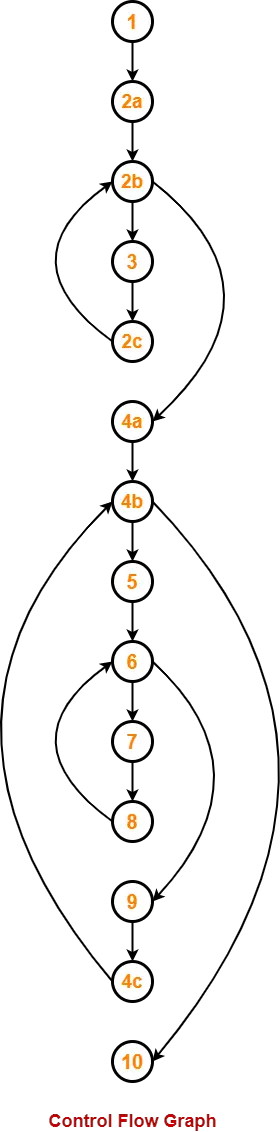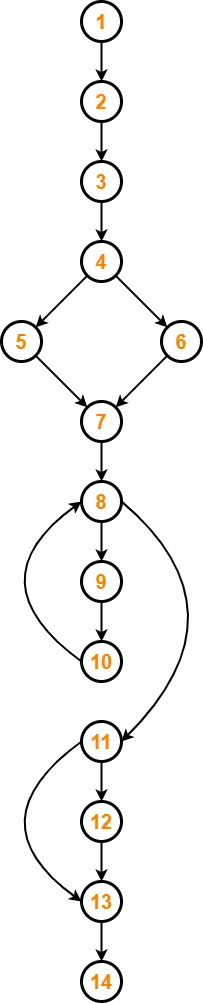# Cyclomatic Complexity | Calculation | Examples

## Cyclomatic Complexity-

Cyclomatic Complexity may be defined as-

• It is a software metric that measures the logical complexity of the program code.
• It counts the number of decisions in the given program code.
• It measures the number of linearly independent paths through the program code.

Cyclomatic complexity indicates several information about the program code-

 Cyclomatic Complexity Meaning 1 – 10 Structured and Well Written Code High Testability Less Cost and Effort 10 – 20 Complex Code Medium Testability Medium Cost and Effort 20 – 40 Very Complex Code Low Testability High Cost and Effort > 40 Highly Complex Code Not at all Testable Very High Cost and Effort

## Importance of Cyclomatic Complexity-

• It helps in determining the software quality.
• It is an important indicator of program code’s readability, maintainability and portability.
• It helps the developers and testers to determine independent path executions.
• It helps to focus more on the uncovered paths.
• It evaluates the risk associated with the application or program.
• It provides assurance to the developers that all the paths have been tested at least once.

## Properties of Cyclomatic Complexity-

• It is the maximum number of independent paths through the program code.
• It depends only on the number of decisions in the program code.
• Insertion or deletion of functional statements from the code does not affect its cyclomatic complexity.
• It is always greater than or equal to 1.

## Calculating Cyclomatic Complexity-

Cyclomatic complexity is calculated using the control flow representation of the program code.

In control flow representation of the program code,

• Nodes represent parts of the code having no branches.
• Edges represent possible control flow transfers during program execution

There are 3 commonly used methods for calculating the cyclomatic complexity-

### Method-01:

Cyclomatic Complexity = Total number of closed regions in the control flow graph + 1

### Method-02:

Cyclomatic Complexity = E – N + 2

Here-

• E = Total number of edges in the control flow graph
• N = Total number of nodes in the control flow graph

### Method-03:

Cyclomatic Complexity = P + 1

Here,

P = Total number of predicate nodes contained in the control flow graph

## Note-

• Predicate nodes are the conditional nodes.
• They give rise to two branches in the control flow graph.

## Problem-01:

Calculate cyclomatic complexity for the given code-

```IF A = 354
THEN IF B > C
THEN A = B
ELSE A = C
END IF
END IF
PRINT A```

## Solution-

We draw the following control flow graph for the given code-Using the above control flow graph, the cyclomatic complexity may be calculated as-

### Method-01:

Cyclomatic Complexity

= Total number of closed regions in the control flow graph + 1

= 2 + 1

= 3

### Method-02:

Cyclomatic Complexity

= E – N + 2

= 8 – 7 + 2

= 3

### Method-03:

Cyclomatic Complexity

= P + 1

= 2 + 1

= 3

## Problem-02:

Calculate cyclomatic complexity for the given code-

```{ int i, j, k;
for (i=0 ; i<=N ; i++)
p[i] = 1;
for (i=2 ; i<=N ; i++)
{
k = p[i]; j=1;
while (a[p[j-1]] > a[k] {
p[j] = p[j-1];
j--;
}
p[j]=k;
}```

## Solution-

We draw the following control flow graph for the given code-Using the above control flow graph, the cyclomatic complexity may be calculated as-

### Method-01:

Cyclomatic Complexity

= Total number of closed regions in the control flow graph + 1

= 3 + 1

= 4

### Method-02:

Cyclomatic Complexity

= E – N + 2

= 16 – 14 + 2

= 4

### Method-03:

Cyclomatic Complexity

= P + 1

= 3 + 1

= 4

## Problem-03:

Calculate cyclomatic complexity for the given code-

```begin int x, y, power;
float z;
input(x, y);
if(y<0)
power = -y;
else power = y;
z=1;
while(power!=0)
{    z=z*x;
power=power-1;
} if(y<0)
z=1/z;
output(z);
end```

## Solution-

We draw the following control flow graph for the given code-Using the above control flow graph, the cyclomatic complexity may be calculated as-

### Method-01:

Cyclomatic Complexity

= Total number of closed regions in the control flow graph + 1

= 3 + 1

= 4

### Method-02:

Cyclomatic Complexity

= E – N + 2

= 16 – 14 + 2

= 4

### Method-03:

Cyclomatic Complexity

= P + 1

= 3 + 1

= 4

To gain better understanding about Cyclomatic Complexity,

Watch this Video Lecture

Next Article- Cause Effect Graph Technique

Get more notes and other study material of Software Engineering.

Watch video lectures by visiting our YouTube channel LearnVidFun.

SummaryArticle Name
Cyclomatic Complexity | Calculation | Examples
Description
Cyclomatic Complexity is a software metric that measures the logical complexity of the program code. Cyclomatic Complexity is calculated using the formula E-N+2. Cyclomatic Complexity Calculation Examples.
Author
Publisher Name
Gate Vidyalay
Publisher Logo